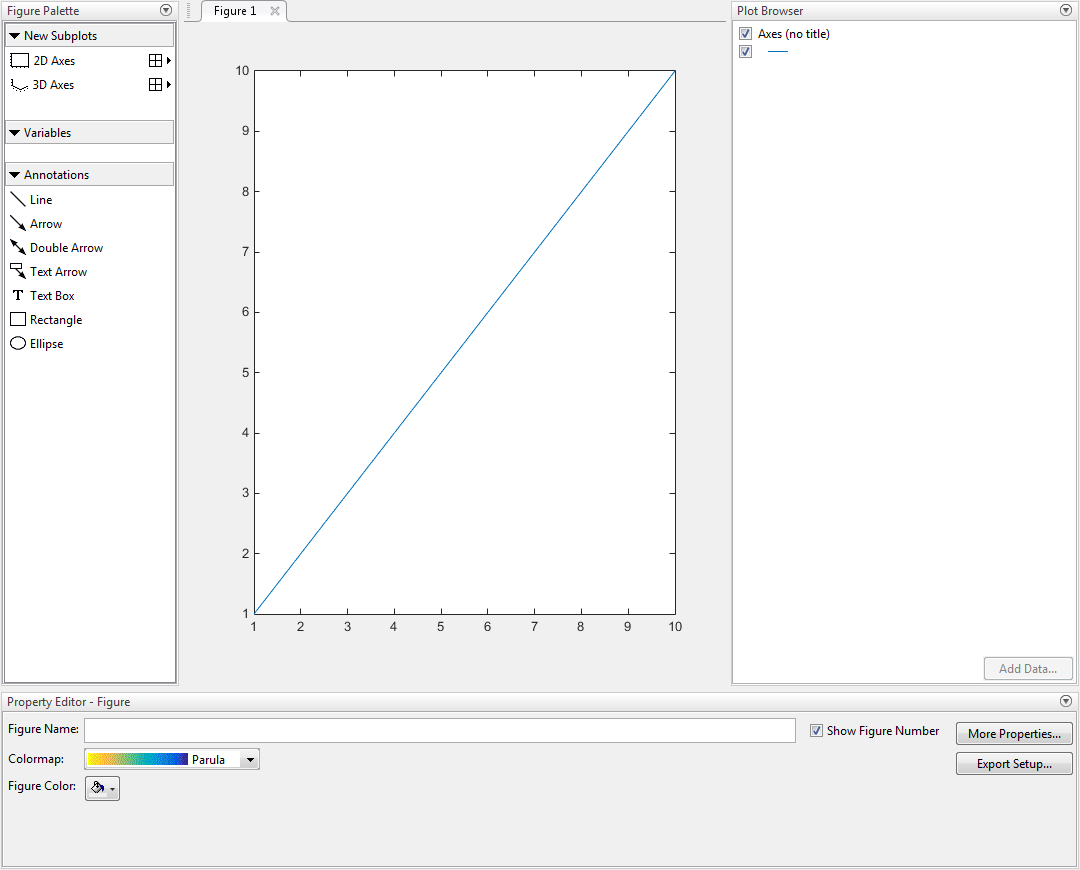# plottools

Show or hide plot tools

## Syntax

```plottools('on') plottools('off') plottools('toggle') plottools plottools(figure_handle,...) plottools(...,'tool') ```

## Description

`plottools('on')` displays the Figure Palette, Plot Browser, and Property Editor on the current figure, configured as you last used them.

`plottools('off')` hides the Figure Palette, Plot Browser, and Property Editor on the current figure.

`plottools('toggle')` toggles the visibility of the Figure Palette, Plot Browser, and Property Editor on the current figure.

`plottools` is the same as `plottools('on')`.

`plottools(figure_handle,...)` displays or hides the plot tools on the specified figure instead of on the current figure.

`plottools(...,'tool')` operates on the specified tool only. `'tool'` can be one of these values:

• `'figurepalette'`

• `'plotbrowser'`

• `'propertyeditor'`

Note

The first time you open the plotting tools, all three of them appear, grouped around the current figure as shown above. If you close, move, or undock any of the tools, MATLAB® remembers the configuration you left them in and restores it when you invoke the tools for subsequent figures, both within and across MATLAB sessions.

## Examples

### Open Plot Tools

Create a line plot and open the plot tools.

```plot(1:10); plottools('on')```## Alternatives

Select the Figure Palette, Plot Browser, and Property Editor tools from the figure's View menu.